## NCERT Solutions for Class 10 Maths Chapter 5 Arithmetic Progressions Ex 5.4

NCERT Solutions for Class 10 Maths Chapter 5 Arithmetic Progressions Ex 5.4 are part of NCERT Solutions for Class 10 Maths. Here we have given NCERT Solutions for Class 10 Maths Chapter 5 Arithmetic Progressions Ex 5.4.

 Board CBSE Textbook NCERT Class Class 10 Subject Maths Chapter Chapter 5 Chapter Name Arithmetic Progressions Exercise Ex 5.4 Number of Questions Solved 5 Category NCERT Solutions

## NCERT Solutions for Class 10 Maths Chapter 5 Arithmetic Progressions Ex 5.4

Ex 5.4 Class 10 Question 1.
Which term of the AP: 121, 117. 113, ….., is its first negative term?
Solution:Ex 5.4 Class 10 NCERT Solutions Question 2.
The sum of the third term and the seventh term of an AP is 6 and their product is 8. Fibd the sum of first sixteen terms of the AP?
Solution: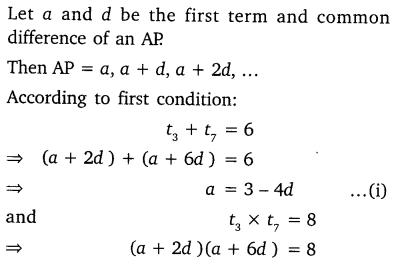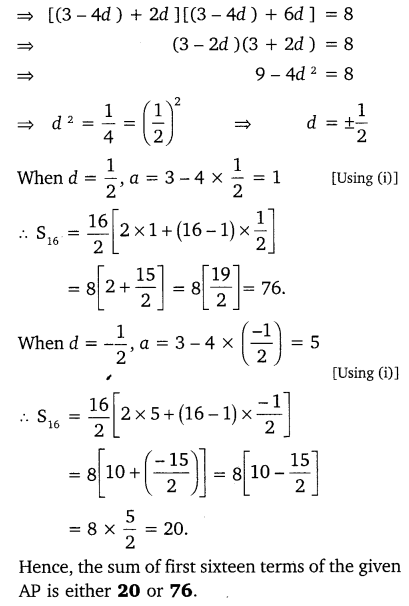Class 10 Ex 5.4 Question 3.
A ladder has rungs 25 cm apart. The rungs decrease uniformly in length from 45 cm at the bottom to 25 at the top. If the top and the bottom rungs are $${ 2 }\frac { 1 }{ 2 } m$$ apart, what is the length of the wood required for the rungs?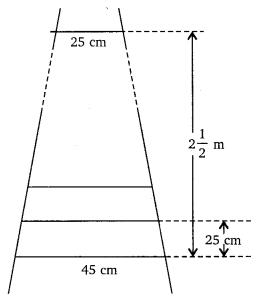Solution:Ex 5.4 Class 10 Question 4.
The houses of a row are numbered consecutively from 1 to 49. Show that there is value of x such that the sum of the numbers of the houses preceding the house numbered x is equal to the sum of the numbers of the houses following it. Find this value of x.
Solution: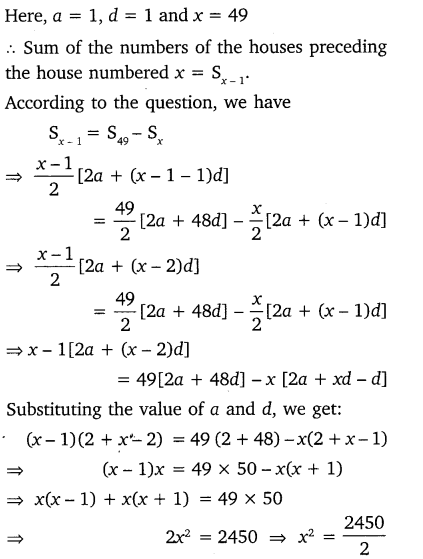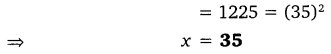Ex 5.4 Class 10 Question 5.
A small terrace at a football ground comprises of 15 steps each of which is 50  m long and built of solid concrete. Each step has a rise of $$\frac { 1 }{ 2 } m$$ and a tread of $$\frac { 1 }{ 2 } m$$. Calculate the total volume of concrete required to build the terrace.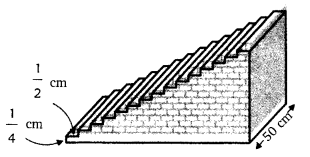Solution: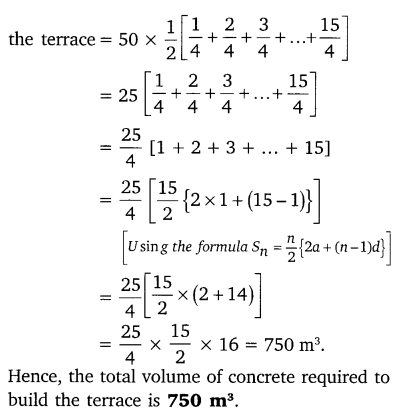We hope the NCERT Solutions for Class 10 Maths Chapter 5 Arithmetic Progressions Ex 5.4, help you. If you have any query regarding NCERT Solutions for Class 10 Maths Chapter 5 Arithmetic Progressions Ex 5.4, drop a comment below and we will get back to you at the earliest.